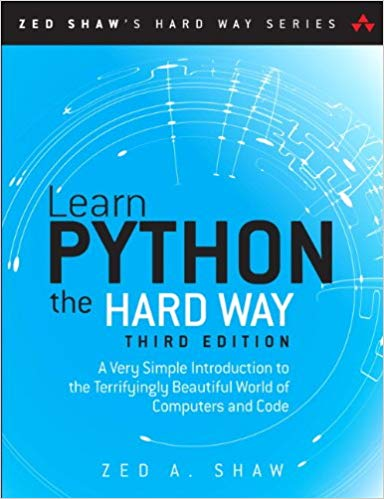## 5 Ways to Check if a String is Integer in Python

A lot of times, while doing some projects or maybe simple programming,

## Python Max Int | What’s the Maximum Value of int Data Type in Python

As 70% of the folks who start learning python come from C,

## Numpy Divide | How to Use Numpy.divide() Function in Python

The Numpy divide function is a part of numpy arithmetic operations. There

## Numpy Multiply | How to Use Numpy.multiply() Function in Python

The Numpy multiply function is a part of numpy arithmetic operations. There

The Numpy add function is a part of numpy arithmetic operations. There

## Numpy Subtract | How to Use Numpy.subtract() Function in Python

The Numpy subtract function is a part of numpy arithmetic operations. There

## Why Should You Learn Python?

### Reason 1: Python is versatile

Just to name a few, Python is used in Data Mining, Data Science, AI, Machine Learning, Web Development, Web Frameworks, Embedded Systems, Graphic Design applications, Gaming, Network development, Product development, Rapid Application Development, Testing, Automation, the list goes on.

### Reason 2: Python’s Popularity & High Salary

Python engineers have some of the highest salaries in the industry. The average Python Developer salary in the United States is approximately \$116,028 per year.

### Reason 3: simple & easy to learn

Python language is expressive and productive. So you can create solutions quickly and others can understand it easily. One of the fundamental ideas behind Python is to facilitate an easily readable code. The syntax of Python is simple, clean and easy to understand. Unlike many other programming languages, its code is relatively easy to read as it resembles a part of everyday English that we speak.

# Join Python Pool Community

## Books We Recommend For Beginners

While there are tons of Python Courses Online, learning Python from a book is still one of the best ways to greatly improve your python skills and knowledge.### Learn Python the Hard Way

Zed A. Shaw’s book may not have the most appealing title, but it can certainly equip you with the skills you need to be an excellent Python developer.### Python Crash Course

This wonderful book helps you to write programs with a thorough introduction, solving problems and generate the results in less time.### Automate the Boring Stuff with Python

You’ll learn how to use Python to write programs that do in minutes what would take you hours to do by hand-no prior programming experience required.